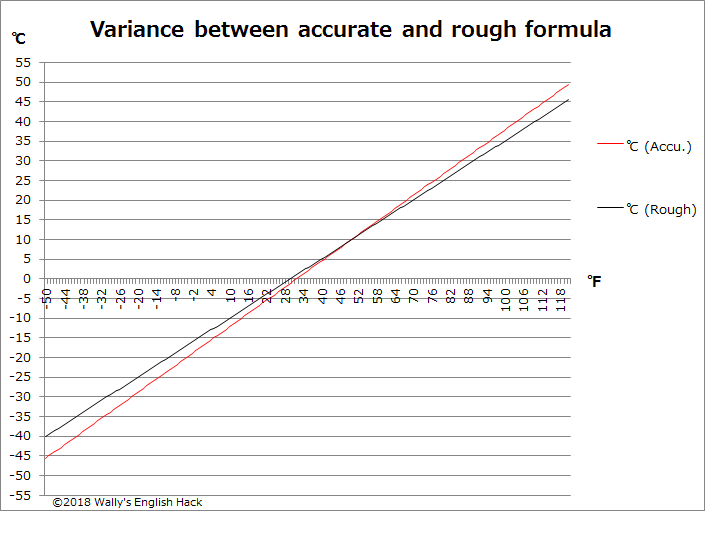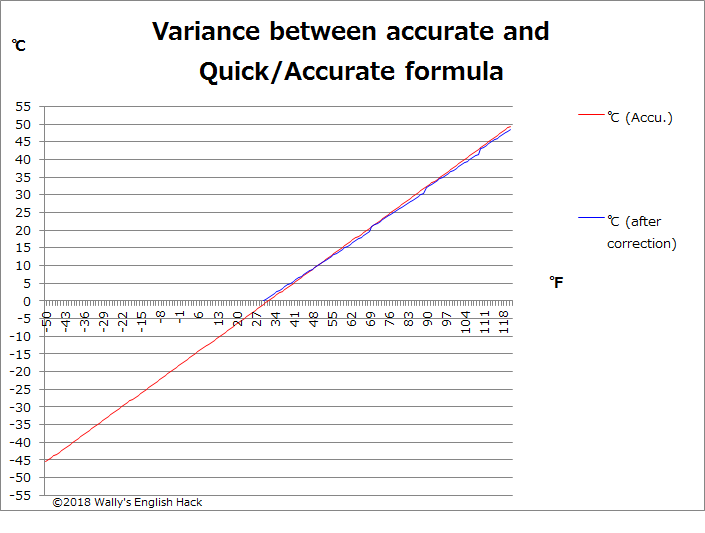# How to convert Fahrenheit to Celsius quickly AND accurately

スポンサーリンク

This article explains how to convert degree Fahrenheit to degree Celsius quickly AND accurately. There are many websites which introduce quick way but most of them lack accuracy, so I hope you will like this one!

If you are travelling outside the US (or reading some science article from overseas), the chances are that you will most likely encounter degree Celsius as temperature scale, not degree Fahrenheit. Note that beside the US, only the Bahamas, Palau, Cayman Islands, Liberia and a few more countries use Fahrenheit, and all the rest of the world are using Celsius.

You would imagine 35 degrees Celsius in Tokyo is quite cold, when actually it is a nasty hot day. So let’s figure out how you can quickly convert these scale.

スポンサーリンク

## The basics

The degree Celsius scale is based on the temperature which water freezes and evaporates. The freezing point is set as 0 degree Celsius, and the boiling point is set as 100 degrees Celsius. So there are 100 steps between the freezing point and the boiling point. Sounds quite straight forward, right?

The freezing point and boiling point will be 32 degrees and 212 degrees in Fahrenheit scale respectively. There are 180 scale steps in between.## Conversion formula

Before we move on to the quick and dirty ways to converting the scales, please let me recap the formal conversion formula.

In order to convert the Fahrenheit scale to the Celsius scale, first you need to subtract 32 from the Fahrenheit temperature, to match the starting point.

Then, as you saw in the previous section, 1 degree in Fahrenheit is 1.8 times greater than Celsius, so divide the value with 1.8. This will get you the degree Celsius value.

Celsius = (Fahrenheit-32) / 1.8

For instance, 64 degrees Fahrenheit will be (64-32)/1.8=17.8 degrees Celsius.

 ℉ ℃ -459.67 -273.15 -50 -45.6 -40 -40.0 -30 -34.4 -20 -28.9 -10 -23.3 0 -17.8 10 -12.2 20 -6.7 30 -1.1 32 0.0 40 4.4 50 10.0 60 15.6 70 21.1 80 26.7 90 32.2 100 37.8 110 43.3 120 48.9 212 100.0

## Quick conversion formula

Having said so, it is not always easy to calculate Fahrenheit minus 32 and dividing by 1.8 in your head. The quick and dirty way to avoid this tricky calculation is to subtract 30 instead of 32, then divide by 2 not 1.8. This will give you fairly accurate result.

Celsius(approx.)=(F-30)/2

For example, 64 degrees Fahrenheit will be (64-30)/2=18 degrees Celsius.

The accurate conversion is 17.8 degrees, so you can see this is pretty accurate.

## Quick AND accurate formula

Subtracting 30 and then dividing by 2 is a well-known method of quick conversion. The issue is that the greater or lower the Fahrenheit degree becomes, the more variance you get.

Example;

50 degrees F = 10 degrees C.   Rough conversion : 10 degrees C.  Variance : 0

80 degrees F = 26.7 degrees C.   Rough conversion : 25 degrees C.  Variance : 1.7

120 degrees F = 48.9 degrees C.   Rough conversion : 45 degrees C.  Variance : 3.9

You can visually see how the variance becomes larger in the chart below.This is where my secret adjustment method comes in play. The method to convert Fahrenheit to Celsius quick AND accurate is;

After the rough calculation, take the number at the tens place and subtract 1. Add that number to the original rough estimate.

Example:

Rough estimation of 90 degrees Fahrenheit = (90-30)/2 = 30 degrees Celsius

Secret sauce : Subtract 1 from 3, which was taken from the tens place = 2

30+2=32 degrees Celsius

The accurate Celsius value of 90 degrees Fahrenheit is 32.2 degrees, so you can see this method gives you pretty good accuracy of conversion.

Other examples

80 degrees F = 26.7 degrees C.   Quick and accurate conversion : 25+(2-1)=26 degrees F. Variance : 0.7 degrees

120 degrees F = 48.9 degrees C.   Quick and accurate conversion : 45+(4-1)=48 degrees F. Variance : 0.9 degrees

Note that this method does not apply to temperatures below the freezing point.

The variance after adding the secret sauce## Where Fahrenheit meets Celsius

By the way, minus 40 degrees is the point where Fahrenheit and Celsius scale matches. You probably won’t think such a cold temperature has nothing to do with you, but my friend who lives in Canada says it can go this low during Winter!

## Postface

My name is Wally, thank you very much for visiting my blog website which introduces American English slang, idioms and life hack tips to Japanese readers. This article is an English translation of the original “How to convert Fahrenheit to Celsius quickly AND accurately” written in Japanese. If you are ever interested in the Japanese article, please visit here!

スポンサーリンク
シェアするWallyの英語(と中国語)ハック

## コメント

1. […] アメリカ人の読者に向けて、この記事の英語版も書きました。ご興味のある方はこちらの記事をどうぞ！ […]